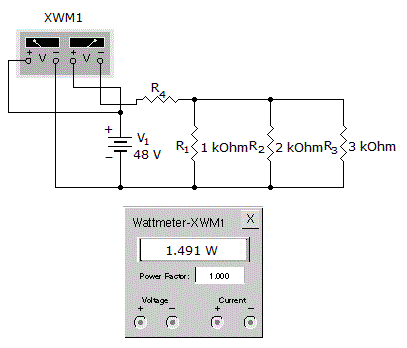# Electronics - Parallel Circuits - Discussion

Discussion Forum : Parallel Circuits - General Questions (Q.No. 37)
37.
What is the total resistance?1.045 k1.545 k2.045 k2.545 kExplanation:
No answer description is available. Let's discuss.
Discussion:
6 comments Page 1 of 1.

@ Barun
As per Anshul said,

P=V^2/R

hence R=1.545

As R=(1/R1)+(1/R2)+(1/R3)+R4

(1/R1)+(1/R2)+(1/R3)=0.545K

That's why R4=1K

Power as seen on wattmeter = 1.491W

Voltage =48V

As we know P=V^2/R

By this relation we get R=1.545 Kohm

In this power is given so R4 value not requried
R=P/V^2
=1494/48*48
=1.54 ohms

Ranjith said:   10 years ago
P = V^2/R.

R = V^2/P.

= (48*48)/1.491.

= 1.545 kohm.

Barun singh said:   1 decade ago
The value of R4 is not give then how you take it 1k.

Ajay bhardwaj said:   9 years ago
@Jaggubai.

From where P/V= 1.494 comes.